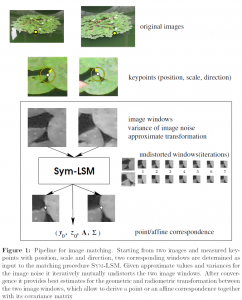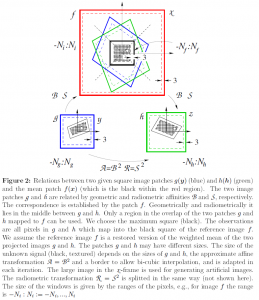# Symmetric Least Squares Matching

Least squares matching (LSM) takes two images as input and determines the mutual geometric and radiometric distortion. We assume the images are square – not necessarily of the same size – and black and white. The distortion is modelled as a geometric transformation with six parameters and a radiometric affinity with two parameters. Exchanging the two images leads to the inverse affinities, which result from the symmetric structure of the internal representation of the model. The noise variance of the individual pixels may be different. The resulting parameters are provided together with their covariance matrix.

## Example## Mathematical formulationMatching_Sym_LSM.zip

## The main routine

The main routines is

LSM_62_sym_main.m

It takes as input

• the two images
• the noise variances
• approximate values for the geometric and radiometric parameters
• and further control parameters

It provides

• the eight estimated parameters of the transformations
• the estimated variance factor
• and further useful indicators

Since Matlab is slow with loops, we provide a second routine

LSM_62_sym_warp_main.m

It has the same input-output scheme, but differs in speed. While LSM_62_sym_main.m uses a loop over all pixels for building the design/Jacobian matrix, the routine LSM_62_sym_warp_main.m exploits the Matlab-internal function imtransform.m.

## The demo routines

There are four demo routines:

1. demo_LSM_small.m
2. demo_LSM_medium.m
3. demo_LSM_simulated.m
4. demo_LSM_image_pairs.m

1. The demo routine demo_LSM_small.m shows the most simple form of using of the main routine, here LSM_62_sym_warp_main.m. The required input data are loaded from file. The routine assumes the approximate values for the geometric transformation is given. Furthermore, the noise variance functions vg and vh are assumed to be provided, e.g. determined from a representative image using the routine noise_standard_deviation_estimation.m. The input images, the noise standard deviations and the change of the estimated image windows are shown in figures.

2. The demo routine demo_LSM_medium.m shows how the noise variance estimation is integrated into the matching process. Again the required input data are loaded from file. Here these are the two complete images together with two corresponding Lowe-keypoints (coordinates, scale, direction). These are used to define the window size and the approximate values. The noise variance functions vg and vh are determined automatically from the area around the keypoints. First, the input images with the keypoints are shown. When zooming into the keypoints the centre and the direction vector fixing the scale and the direction can be seen. Further figures show the selected windows, the noise standard deviations and the change of the estimated image windows.

3. The demo routine demo_LSM_simulated.m is meant to check the correctness of the implementation based on simulated data. It allows to monitor the individual iterations for a single case, or to statistically test, whether the resultant parameters and their covariance matrix are coherent with the theoretical values, the true values of the parameters and the theoretical covariance matrix derived by the estimation procedure (Cramer-Rao bound).

4. The demo routine demo_LSM_image_pairs.m allows to apply Sym-LSM to real data. Approximate values can be provided interactively, or – later – read from file. This refers to the centre of the two windows, their individual scale and their individual main direction, following the notion of scale and direction of Lowe’s keypoints.

## The folder structure

The folder contains

• three demo-routines
demo_LSM_small.m
demo_LSM_medium.m
demo_LSM_simulated.m
demo_LSM_image_pairs.m
• three subfolders
doc: the documentation
example_data: data for testing the software
src: Matlab-source code

The documentation contains a description of the underlying model, the method and the principle of the algorithm (Sym-LSM-symmetric-least-squares-matching.pdf).

Using the software m2html.m (https://www.artefact.tk/software/matlab/m2html/) all routines are documented together with the function-hierarchy. The root-file is doc/html/MENU.html.

## References

If you use this software for a publications, please refer to:

Barath, D., M. Polic, W. Förstner, T. Sattler, T. Pajdla, and Z. Kukelova (2020a). Making Affine Correspondences Work in Camera Geometry Computation – with Supplement. In Proc. of ECCV. with Supplement

Förstner, W. (2020). Symmetric Least Squares Matching — Sym-LSM. Internal report. Institute for Geodesy and Geoinformation, Professorship of Photogrammetry, University of Bonn

## Author

Wolfgang Förstner
e-mail: wf@nullipb.uni-bonn.de
home-page: www.ipb.uni-bonn.de

University Bonn
Institute of Geodesy and Geoinformation
Department of Photogrammetry
Nussallee 15, D-53115 Bonn